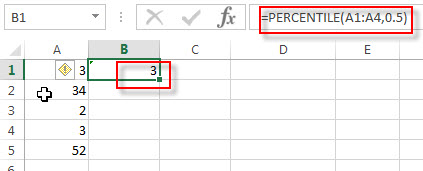## Excel PERCENTILE Function

This post will guide you how to use the PERCENTILE function with syntax and examples in Microsoft excel.

### Description

The Excel PERCENTILE function returns the kth percentile from a supplied range of values. So you can use the PERCENTILE function to determine the 30% percentile in a range in Excel. This function has been replaced by the PERCENTILE.INC function in Excel 2010.

The PERCENTILE function is a build-in function in Microsoft Excel and it is categorized as a Statistical Function.

The PERCENTILE function is available in Excel 2016, Excel 2013, Excel 2010, Excel 2007, Excel 2003, Excel XP, Excel 2000, Excel 2011 for Mac.

### Syntax

The syntax of the PERCENTILE function is as below:

`= PERCENTILE (array,k)`

Where the PERCENTILE function arguments are:

• array – This is a required argument.  A range or array or cell reference that you want to get the kth percentile.
• K – This is a required argument. The percentile value. And it can be a value between 0 and 1.

Note:

• If K argument is non-numeric, the PERCENTILE function will return the #VALUE! Error.
• If K is small than 0 or greater than 1, the PERCENTILE function will return the #NUM! Error.
• If k is not a multiple of 1/(n – 1), PERCENTILE interpolates to determine the value at the k-th percentile.

### Excel PERCENTILE Function Examples

The below examples will show you how to use Excel PERCENTILE Function to get the k-th percentile of values for a set of data.

Example 1:  to get the 50th percentile of the values in the range A1:A4, using the following formula:

`= PERCENTILE(A1:A4,0.5)`